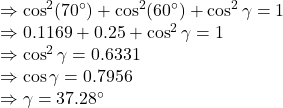## 30 unit If the value of an angle with the x-axis of a vector is 70 °, the value of the angle with the y axis is 60, what is the value of the

Question

30 unit If the value of an angle with the x-axis of a vector is 70 °, the value of the angle with the y axis is 60, what is the value of the angle with the z-axis?

in progress 0
2 weeks 2021-08-30T18:40:41+00:00 1 Answers 0 views 0

1. Answer: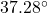Explanation:

Given

Angle made with the x-axis is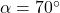Angle made with the y-axis is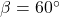Suppose angle made with z-axis is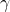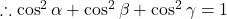Inserting values